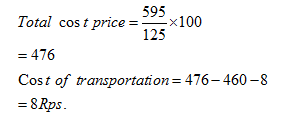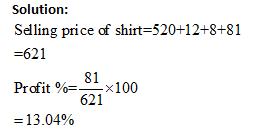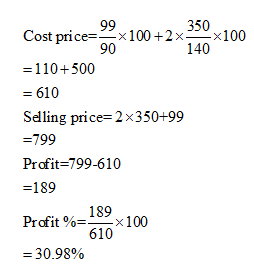New Students Offer - Use Code HELLO

# Missing DI Practice Set for RBI Grade B Part-1

Directions (Q1-5) Study the following data carefully and answer the questions:
The percentage profit is given on total cost price.
Total Cost= Cost of production + Cost of packaging + Cost of transportation

Name of Goods Cost of production (1 piece) Cost of packaging Cost of transportation Profit/Loss Selling price         (1 piece) Percentage of Profit/ loss
Shirt 520 12 8 81 - -
Skirt 220 10 - - 350 40%
Top 460 8 - - 595 -
Socks (1 pair) - - 5 - 99 10% Loss
Jeans 830 - 20 50 - -

Q1. If the percentage of profit of top is 25%, then what is its cost of transportation?
A. 20
B. 16
C. 8
D. 12
E. None of these

Q2. What is the difference between the cost price of socks and jeans. If the cost of packaging and transportation is zero for both.
A. 810
B. 720
C. 750
D. 790
E. None of these

Q3. What is the profit percentage of a shirt on selling price?
A. 12%
B. 14.11%
C. 15%
D. 13.04%
E. None of these

Q4. What is the ratio between the total cost price of shirt and selling price of top?
A. 108:120
B. 110:119
C. 108:119
D. 119:108
E. None of these

Q5. If 2 skirt and 1 pair of socks are sold then find the percentage of total profit/loss on cost price?
A. 15.20%
B. 20.15%
C. 32%
D. 30.98%
E. 24.72%

## Solution:

#### 1. Ans. C.#### 2. Ans. B.#### 3. Ans. D.#### 4. Ans. C#### 5. Ans. D.